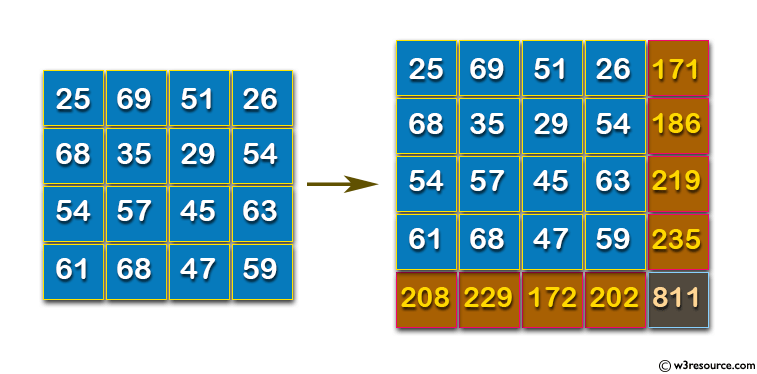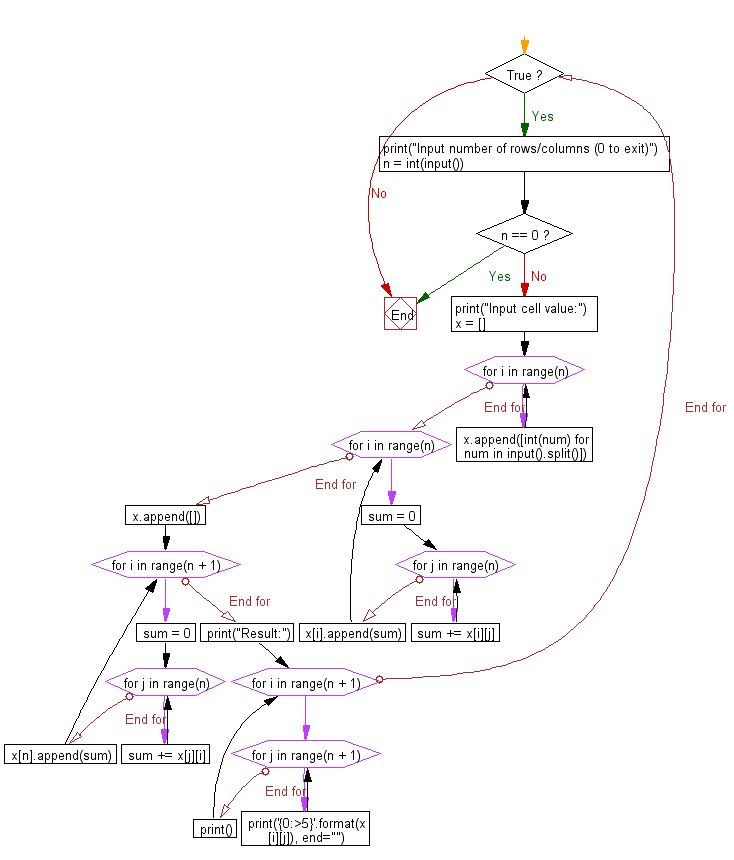﻿ Python: Adds up columns and rows of given table as shown in the specified figure - w3resource# Python: Adds up columns and rows of given table as shown in the specified figure

## Python Basic - 1: Exercise-63 with Solution

Write a Python program which adds up columns and rows of given table as shown in the following figure:

Pictorial Presentation:n (the size of row and column of the given table)
1st row of the table
2nd row of the table
:
:
n th row of the table
The input ends with a line consisting of a single 0.
Output:
For each dataset, print the table with sum of rows and columns.

Sample Solution:

Python Code:

``````while True:
print("Input number of rows/columns (0 to exit)")
n = int(input())
if n == 0:
break
print("Input cell value:")
x = []
for i in range(n):
x.append([int(num) for num in input().split()])

for i in range(n):
sum = 0
for j in range(n):
sum += x[i][j]
x[i].append(sum)

x.append([])
for i in range(n + 1):
sum = 0
for j in range(n):
sum += x[j][i]
x[n].append(sum)
print("Result:")
for i in range(n + 1):
for j in range(n + 1):
print('{0:>5}'.format(x[i][j]), end="")
print()
``````

Sample Output:

```Input number of rows/columns (0 to exit)
4
Input cell value:
25 69 51 26
68 35 29 54
54 57 45 63
61 68 47 59
Result:
25   69   51   26  171
68   35   29   54  186
54   57   45   63  219
61   68   47   59  235
208  229  172  202  811
Input number of rows/columns (0 to exit)
```

Flowchart:Python Code Editor:

Have another way to solve this solution? Contribute your code (and comments) through Disqus.

What is the difficulty level of this exercise?

Test your Programming skills with w3resource's quiz.

﻿

## Python: Tips of the Day

Iterating over dictionaries using 'for' loops:

I am a bit puzzled by the following code: d = {'x': 1, 'y': 2, 'z': 3} for key in d: print key, 'corresponds to', d[key] What I don't understand is the key portion. How does Python recognize ...

key is just a variable name.

```for key in d:
```

For Python 3.x:

```for key, value in d.items():
```

For Python 2.x:

```for key, value in d.iteritems():
```

To test for yourself, change the word key to poop.

In Python 3.x, iteritems() was replaced with simply items(), which returns a set-like view backed by the dict, like iteritems() but even better. This is also available in 2.7 as viewitems().

The operation items() will work for both 2 and 3, but in 2 it will return a list of the dictionary's (key, value) pairs, which will not reflect changes to the dict that happen after the items() call. If you want the 2.x behavior in 3.x, you can call list(d.items()).

Ref: https://bit.ly/37dm0Qo